﻿ 不同分布下均值差的检验问题

# 不同分布下均值差的检验问题Research on the Test of Mean Value Difference under Different Distributions

Abstract: In many statistical problems, there will be nuisance parameters. In the case of small samples, the traditional frequency school cannot give a better method. However, the acquisition of large samples is often costly and even impossible. The generalized inference solves this kind of inspection problems very well. Generalized inference is a statistical inference method based on generalized test variables and generalized pivotal quantity. With the development of information technology and data analysis, generalized inference is playing its good performance and has been widely used. This article examines some problems of significance for one-sided hypotheses, on the basis of generalized pivotal quantity, generalized p value is given and the confidence interval of the interest parameter is given by the one-to-one correspondence between the hypothesis test and the confidence interval.

1. 引言

2. 预备知识

2.1. 广义检验变量和广义P值

1) 对给定的x和 $\eta =\left({\theta }_{0},\delta \right)$$T=\left(X;x,\eta \right)$ 的分布函数与讨厌参数 $\delta$ 无关。

2) 观测值 $T=\left(x;x,\eta \right)$$\eta$ 无关。

3) 对给定的x和 $P\left(T\left(X;x,\eta \right)\ge T\left(x;x,\eta \right)|x,\eta \right)$ 关于 $\theta$ 非减(或非增)。

${H}_{0}:\theta \le {\theta }_{0}$ , ${H}_{1}:\theta >{\theta }_{0}$ (1)

$T=\left(X;x,\eta \right)$ 关于 $\theta$ 非减，则关于检验问题(1)的广义p值为

$\underset{\theta \le {\theta }_{0}}{\mathrm{sup}}P\left(T\left(X;x,\eta \right)\ge t\left(x\right)|\theta ={\theta }_{0}\right)$

$T=\left(X;x,\eta \right)$ 关于 $\theta$ 非增，则关于检验问题(1)的广义p值为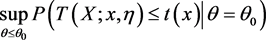2.2. 广义枢轴量和广义置信区间

1) 对给定的x， $R\left(X;x,\eta \right)$ 的分布与未知参数 $\eta =\left(\theta ,\delta \right)$ 无关。

2) 观测值 $r=R\left(x;x,\eta \right)$ 与讨厌参数 $\delta$ 无关。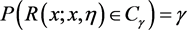,

${\Theta }_{\gamma }=\left\{\theta |R\left(x;x,\eta \right)\in {C}_{\gamma }\right\}$ ,

$T+R=g\left(\theta \right)$ ，其中 $g\left(\theta \right)$ 为兴趣参数的函数，因此可以通过构造广义枢轴量的方法来进行假设检验，且其相应的广义p值可以通过二者的关系计算得到。

2.3. Fiducial广义枢轴量

$R\left(X;x,\eta \right)$ 是关于 $X,x$$\eta$ 的参数，其中 $\eta =\left(\theta ,\delta \right)$$\theta$ 是兴趣参数， $\delta$ 是讨厌参数，并且满足以下条件：

1) 对给定的x， $R\left(X;x,\eta \right)$ 的分布与未知参数 $\eta =\left(\theta ,\delta \right)$ 无关。

2) 观测值 $R\left(x,x,\eta \right)=\theta$

3. 广义枢轴量法在假设检验与置信区间中的应用

3.1. 两正态分布均值差的检验

${X}_{1},{X}_{2},\cdots ,{X}_{m}$${Y}_{1},{Y}_{2},\cdots ,{Y}_{n}$ 是分别来自两正态总体 $N\left({\mu }_{1},{\sigma }_{1}^{2}\right)$$N\left({\mu }_{2},{\sigma }_{2}^{2}\right)$ 的随机样本， ${X}_{i}$${Y}_{j}$$i=1,\cdots ,m$$j=1,\cdots ,n$ 相互独立，其中考虑检验问题：

${H}_{0}:{\mu }_{1}-{\mu }_{2}\le 0$ , ${H}_{1}:{\mu }_{1}-{\mu }_{2}>0$ (2)

$\stackrel{¯}{X}=\frac{{\sum }_{i=1}^{m}{X}_{i}}{m}$$\stackrel{¯}{Y}=\frac{{\sum }_{i=1}^{n}{Y}_{i}}{n}$${S}_{1}^{2}=\frac{{\sum }_{i=1}^{m}{\left({X}_{i}-\stackrel{¯}{X}\right)}^{2}}{m}$${S}_{2}^{2}=\frac{{\sum }_{i=1}^{n}{\left({Y}_{i}-\stackrel{¯}{Y}\right)}^{2}}{n}$

${U}_{1}=\frac{\stackrel{¯}{X}-{\mu }_{1}}{\sqrt{{\sigma }_{1}^{2}/m}}~N\left(0,1\right)$ , ${U}_{2}=\frac{\stackrel{¯}{Y}-{\mu }_{2}}{\sqrt{{\sigma }_{2}^{2}/n}}~N\left(0,1\right)$

${K}_{1}=\frac{m{S}_{1}^{2}}{{\sigma }_{1}^{2}}~{\chi }_{m-1}^{2}$ , ${K}_{2}=\frac{n{S}_{2}^{2}}{{\sigma }_{2}^{2}}~{\chi }_{n-1}^{2}$

$\left(\stackrel{¯}{x},\stackrel{¯}{y},{s}_{1}^{2},{s}_{2}^{2}\right)$$\left(\stackrel{¯}{X},\stackrel{¯}{Y},{S}_{1}^{2},{S}_{2}^{2}\right)$ 的观测值。

$R\left(X,Y,x,y,\eta \right)=\left(\stackrel{¯}{X}-\stackrel{¯}{Y}\right)-\left({U}_{1}\sqrt{{s}_{1}^{2}/{K}_{1}}-{U}_{2}\sqrt{{s}_{2}^{2}/{K}_{2}}\right)$

${r}_{obs}=R\left(x,y,x,y,\eta \right)={\mu }_{1}-{\mu }_{2}$ ，则关于检验问题(2)的广义p值为：

$\begin{array}{c}p=\mathrm{Pr}\left(R\left(X,Y,x,y,\eta \right)\le r\left(x,y,x,y,\eta \right)|{\mu }_{1}-{\mu }_{2}=0\right)\\ =\mathrm{Pr}\left({U}_{1}\sqrt{\frac{{s}_{1}^{2}}{{K}_{1}}}-{U}_{2}\sqrt{\frac{{s}_{2}^{2}}{{K}_{2}}}\le \stackrel{¯}{x}-\stackrel{¯}{y}\right)\\ =\mathrm{Pr}\left(\sqrt{\frac{{s}_{1}^{2}}{m-1}}\frac{{U}_{1}}{\sqrt{{K}_{1}/m-1}}-\sqrt{\frac{{s}_{2}^{2}}{n-1}}\frac{{U}_{2}}{\sqrt{{K}_{2}/n-1}}\le \stackrel{¯}{x}-\stackrel{¯}{y}\right)\\ =\mathrm{Pr}\left(\sqrt{\frac{{s}_{1}^{2}}{m-1}}{T}_{1}-\sqrt{\frac{{s}_{2}^{2}}{n-1}}{T}_{2}\le \stackrel{¯}{x}-\stackrel{¯}{y}\right)\\ =\mathrm{Pr}\left({T}_{1}\le \left(\left(\stackrel{¯}{x}-\stackrel{¯}{y}\right)+\sqrt{\frac{{s}_{2}^{2}}{n-1}}{T}_{2}\right){\left(\frac{{s}_{1}^{2}}{m-1}\right)}^{-\frac{1}{2}}\right)\\ ={E}_{{T}_{2}}\left({F}_{{T}_{1}}\left(\left(\stackrel{¯}{x}-\stackrel{¯}{y}\right)+\sqrt{\frac{{s}_{2}^{2}}{n-1}}{T}_{2}\right){\left(\frac{{s}_{1}^{2}}{m-1}\right)}^{-\frac{1}{2}}\right)\end{array}$

${F}_{{T}_{1}}\left(\cdot \right)$ 是自由度为 $m-1$ 的t分布的分布函数， ${E}_{{T}_{2}}$ 是对 ${T}_{2}$ 来求得。

$\begin{array}{l}P\left(R\left(X,Y,x,y,\eta \right)\ge c\right)\\ ={P}_{r}\left({T}_{1}\ge \left(\stackrel{¯}{x}-\stackrel{¯}{y}+c\right)+\sqrt{\frac{{s}_{2}^{2}}{n-1}}{T}_{2}\right){\left(\frac{{s}_{1}^{2}}{m-1}\right)}^{-\frac{1}{2}}\\ =1-{E}_{{T}_{2}}\left({F}_{{T}_{1}}\left(\left(\stackrel{¯}{x}-\stackrel{¯}{y}+c\right)+\sqrt{\frac{{s}_{2}^{2}}{n-1}}{T}_{2}\right){\left(\frac{{s}_{1}^{2}}{m-1}\right)}^{-\frac{1}{2}}\right)=\gamma \end{array}$

$1-{E}_{{T}_{2}}\left({F}_{{T}_{1}}\left(\left(\stackrel{¯}{x}-\stackrel{¯}{y}+{c}_{\gamma }\right)+\sqrt{\frac{{s}_{2}^{2}}{n-1}}{T}_{2}\right){\left(\frac{{s}_{1}^{2}}{m-1}\right)}^{-\frac{1}{2}}\right)=\gamma$

3.2. 两指数分布均值差的检验

$G\left(\alpha ,\beta \right)$ 表示形状参数为 $\alpha$ ，尺度参数为 $\beta$ 的伽马分布， ${Y}_{1},{Y}_{2},\cdots ,{Y}_{n}$ 是分别来自指数分布总体 $G\left(1,1/{\mu }_{1}\right)$$G\left(1,1/{\mu }_{2}\right)$ 的随机样本，均值分别为 ${\mu }_{1}$${\mu }_{2}$${X}_{i}$${Y}_{j}$$i=1,\cdots ,m$$j=1,\cdots ,n$ 相互独立，考虑检验问题：

${H}_{0}:{\mu }_{1}-{\mu }_{2}\le {\delta }_{0}$ , ${H}_{1}:{\mu }_{1}-{\mu }_{2}>{\delta }_{0}$ (3)

$U=\frac{X}{{\mu }_{1}}~G\left(m,1\right)$$V=\frac{Y}{{\mu }_{2}}~G\left(n,1\right)$

$R\left(X,Y,x,y,\eta \right)=\frac{x}{U}-\frac{y}{V}$ ，其中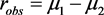，则关于检验问题(3)的广义p值为：

$\begin{array}{c}p=\mathrm{Pr}\left(R\left(X,Y,x,y,\eta \right)\le r\left(x,y,x,y,\eta \right)|{\mu }_{1}-{\mu }_{2}={\delta }_{0}\right)\\ =\mathrm{Pr}\left(\frac{x}{U}-\frac{y}{V}\le {\delta }_{0}\right)=\mathrm{Pr}\left(U\ge \frac{x}{{\delta }_{0}+y/V}{\delta }_{0}\right)=1-{E}_{V}\left({F}_{U}\left(\frac{x}{{\delta }_{0}+y/V}\right)\right)\end{array}$

$\mathrm{Pr}\left(\frac{x}{U}-\frac{y}{V}\ge c\right)=\mathrm{Pr}\left(V\ge \frac{y}{x/U-c}\right)=1-{E}_{U}\left({F}_{V}\left(\frac{y}{x/U-c}\right)\right)=\gamma$

${\mu }_{1}-{\mu }_{2}$ 的置信系数为 $\gamma$ 的置信区间为 $\left({c}_{\gamma },\infty \right)$ ，其中 ${c}_{\gamma }$ 满足：

$1-{E}_{U}\left({F}_{V}\left(\frac{y}{x/U-{c}_{\gamma }}\right)\right)=\gamma$

3.3. 截尾指数分布均值差的检验

${X}_{1},{X}_{2},\cdots ,{X}_{m}$ 来自一截尾指数分布的一个样本，其密度函数为：

$p\left(x;\alpha ,\beta \right)=\left\{\begin{array}{l}\frac{1}{\beta }\left\{-\frac{x-\alpha }{\beta }\right\},\text{\hspace{0.17em}}\text{\hspace{0.17em}}\text{ }x\ge \alpha \\ 0,\text{\hspace{0.17em}}\text{\hspace{0.17em}}\text{\hspace{0.17em}}\text{\hspace{0.17em}}\text{\hspace{0.17em}}\text{\hspace{0.17em}}\text{\hspace{0.17em}}\text{\hspace{0.17em}}\text{\hspace{0.17em}}\text{\hspace{0.17em}}\text{\hspace{0.17em}}\text{\hspace{0.17em}}\text{\hspace{0.17em}}\text{\hspace{0.17em}}\text{\hspace{0.17em}}\text{\hspace{0.17em}}\text{\hspace{0.17em}}\text{\hspace{0.17em}}\text{\hspace{0.17em}}x\le \alpha \end{array}$

${H}_{0}:\alpha +\beta \le {\mu }_{0}$ ,  (4)

$U-\alpha ~G\left(1;n/\beta \right)$ ; 

${Y}_{1}=\frac{2n}{\beta }\left(U-\alpha \right)~{\chi }_{2}^{2}$ ; ${Y}_{2}=\frac{2n}{\beta }V~{\chi }_{2\left(n-1\right)}^{2}$

$R\left(\left(U,V\right);\left(u,v\right),\eta \right)=u-v\frac{{Y}_{1}}{{Y}_{2}}+2nv\frac{1}{{Y}_{2}}$ ，其中 ${r}_{obs}=\alpha +\beta$

$p=\mathrm{Pr}\left(R\left(\left(V,U\right);\left(v,u\right),\theta \right)\le r\left(\left(u,v\right);\left(u,v\right),\theta \right)|\theta ={\mu }_{0}\right)$

$\begin{array}{l}\mathrm{Pr}\left(u-v\frac{{Y}_{1}}{{Y}_{2}}+2nv\frac{1}{{Y}_{2}}\le {\mu }_{0}\right)\\ =\mathrm{Pr}\left(\left({Y}_{1}-2n\right)/{Y}_{2}\ge \left(u-{\mu }_{0}\right)/v\right)\\ ={E}_{{Y}_{1}}\left({\psi }_{{Y}_{2}}\left(\left({Y}_{1}-2n\right){\left(u-{\mu }_{0}\right)}^{-1}\right)\right)\end{array}$

$\begin{array}{l}\mathrm{Pr}\left(u-v\frac{{Y}_{1}}{{Y}_{2}}+2nv\frac{1}{{Y}_{2}}\ge c\right)\\ =\mathrm{Pr}\left(u-v\frac{{Y}_{1}}{{Y}_{2}}+2nv\frac{1}{{Y}_{2}}\ge c\right)\\ =1-{E}_{{Y}_{1}}\left({\psi }_{{Y}_{2}}\left(\left({Y}_{1}-2n\right){\left(u-c\right)}^{-1}\right)\right)=\gamma \end{array}$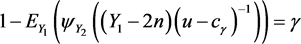3.4. 指数分布定数截断寿命试验均值差的检验

${X}_{1},{X}_{2},\cdots ,{X}_{m}$${Y}_{1},{Y}_{2},\cdots ,{Y}_{n}$ 是代表寿命的随机样本，分别来自指数分布总体 $G\left(1,1/{\mu }_{x}\right)$$G\left(1,1/{\mu }_{y}\right)$ ，其均值分别为 ${\mu }_{x}$${\mu }_{y}$${X}_{i}$${Y}_{j}$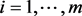$j=1,\cdots ,n$ ，相互独立，同时假设对两总体寿命的观察分

${H}_{0}:{\mu }_{x}-{\mu }_{y}\ge {\delta }_{0}$ , ${H}_{1}:{\mu }_{x}-{\mu }_{y}<{\delta }_{0}$ (5)

${W}_{1}=\frac{2U}{{\mu }_{x}}~{\chi }_{2m}^{2}$ ; ${W}_{2}=\frac{2V}{{\mu }_{y}}~{\chi }_{2n}^{2}$

$R\left(\left(U,V\right);\left(u,v\right),\eta \right)=\frac{2u}{{W}_{1}}-\frac{2v}{{W}_{2}}$ ，其中 ${r}_{obs}={\mu }_{x}-{\mu }_{y}$

$\begin{array}{c}p=\mathrm{Pr}\left(R\left(\left(V,U\right);\left(v,u\right),\eta \right)\ge r\left(\left(u,v\right);\left(u,v\right),\eta \right)|\theta ={\delta }_{0}\right)\\ =\mathrm{Pr}\left(\frac{2u}{{W}_{1}}-\frac{2v}{{W}_{2}}\ge {\delta }_{0}\right)=\mathrm{Pr}\left({W}_{2}\ge \frac{2v}{2u/{W}_{1}-{\delta }_{0}}\right)=1-{E}_{{W}_{1}}\left({F}_{{W}_{2}}\left(\frac{2v}{2u/{W}_{1}-{\delta }_{0}}\right)\right)\end{array}$

$\mathrm{Pr}\left(\frac{2u}{{W}_{1}}-\frac{2v}{{W}_{2}}\le c\right)=1-{E}_{{W}_{2}}\left({F}_{{W}_{1}}\left(\frac{2u}{c+2v/{W}_{2}}\right)\right)=\gamma$

 .

3.5. 关于随机效应模型的检验

${X}_{ij}=\mu +{\alpha }_{i}+{e}_{ij}$ , $i=1,\cdots ,a;j=1,\cdots ,n$ ,

${S}_{1}=\underset{i=1}{\overset{a}{\sum }}\underset{j=1}{\overset{n}{\sum }}{\left({X}_{ij}-{\stackrel{¯}{X}}_{i}\right)}^{2}$ , ${S}_{2}=n\underset{i=1}{\overset{a}{\sum }}{\left({\stackrel{¯}{X}}_{i}-\stackrel{¯}{X}\right)}^{2}$

${H}_{0}:{\sigma }_{\alpha }^{2}\le {\delta }_{0}$ , ${H}_{1}:{\sigma }_{\alpha }^{2}>\delta$ (6)

$U=\frac{{S}_{1}}{{\sigma }_{e}^{2}}~{\chi }_{a\left(n-1\right)}^{2}$ , $V=\frac{{S}_{2}}{{\sigma }_{e}^{2}+n{\sigma }_{\alpha }^{2}}~{\chi }_{a-1}^{2}$

$p={P}_{r}\left(\frac{{s}_{2}}{nV}-\frac{{s}_{1}}{nU}\le \delta \right)={P}_{r}\left(V\ge \frac{{s}_{2}}{{s}_{1}/U+n\delta }\right)=1-{E}_{U}\left({F}_{V}\left(\frac{{s}_{2}}{{s}_{1}/U+n\delta }\right)\right)$ .

$P\left(\frac{{s}_{2}}{nV}-\frac{{s}_{1}}{nU}\ge c\right)={E}_{U}\left({F}_{V}\left(\frac{{s}_{2}}{{s}_{1}/U+nc}\right)\right)=\gamma$

4. 结论

 Tsui, K.W. and Weerahandi, S. (1989) Generalized p-Values in Significance Testing of Hypotheses in the Presence of Nuisance Parameters. Journal of the American Statistical Association, 84, 602-607.
https://doi.org/10.2307/2289949

 Weerahandi, S. (1993) Generalized Confidence Intervals. Journal of the American Statistical Association, 88, 899-905.
https://doi.org/10.1080/01621459.1993.10476355

 牟唯嫣. 广义枢轴量的构造、应用及推广[D]: [博士学位论文]. 北京: 北京理工大学, 2009.

Top